Function Repository Resource:

# MatrixFieldOfValues

Evaluate the boundary curve of the field of values of a matrix

Contributed by: Jan Mangaldan
 ResourceFunction["MatrixFieldOfValues"][m,t] evaluates the boundary curve of the field of values for a numerical square matrix m at t.

## Details

The field of values is also known as the numerical range of a matrix.
The field of values of a square matrix m is the nonempty bounded convex set in the complex plane consisting of all the Rayleigh quotients of m; that is, all the numbers of the form Conjugate[v].m.v, where v is a unit vector.
The unit vector v corresponding to the point returned by ResourceFunction["MatrixFieldOfValues"][m,t] is the eigenvector corresponding to the largest eigenvalue of the Hermitian matrix (g+ConjugateTranspose[g])/2, where g=mExp[t].
SparseArray objects can be used in ResourceFunction["MatrixFieldOfValues"].

## Examples

### Basic Examples (2)

Compute a point on the boundary curve of the field of values for a matrix:

 In:=Out=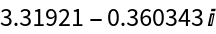A square matrix:

 In:=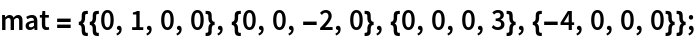Visualize the field of values of the matrix along with its eigenvalues:

 In:=Out=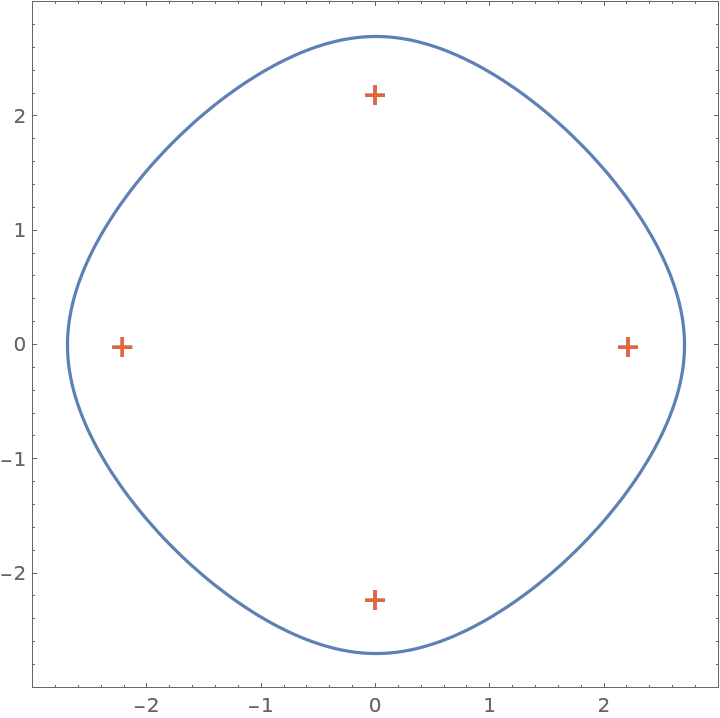### Scope (3)

Evaluate MatrixFieldOfValues for a numerical matrix and a numerical value:

 In:=Out=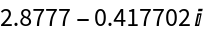MatrixFieldOfValues works for SparseArray objects:

 In:=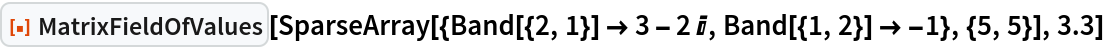Out=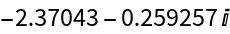MatrixFieldOfValues threads elementwise over lists in the last argument:

 In:=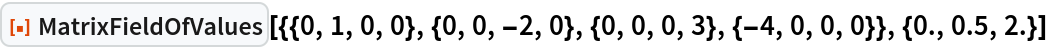Out=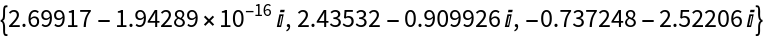### Applications (2)

Visualize the field of values for a large sparse matrix:

 In:=Out=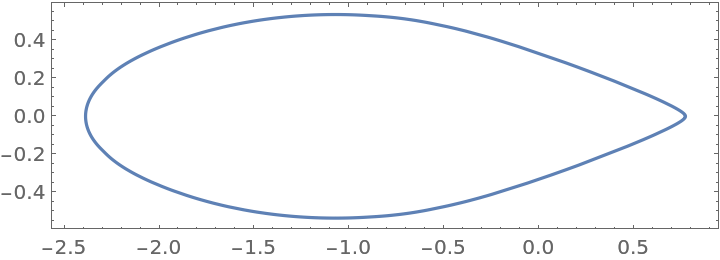Brillhart's cubic:

 In:=Out=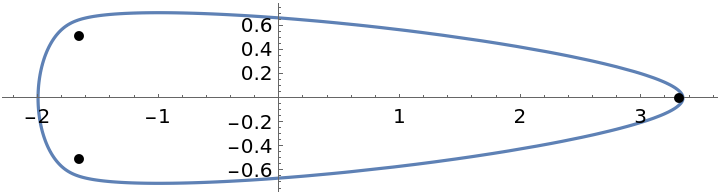### Properties and Relations (3)

The field of values for a 2×2 matrix is bounded by an ellipse:

 In:=Out=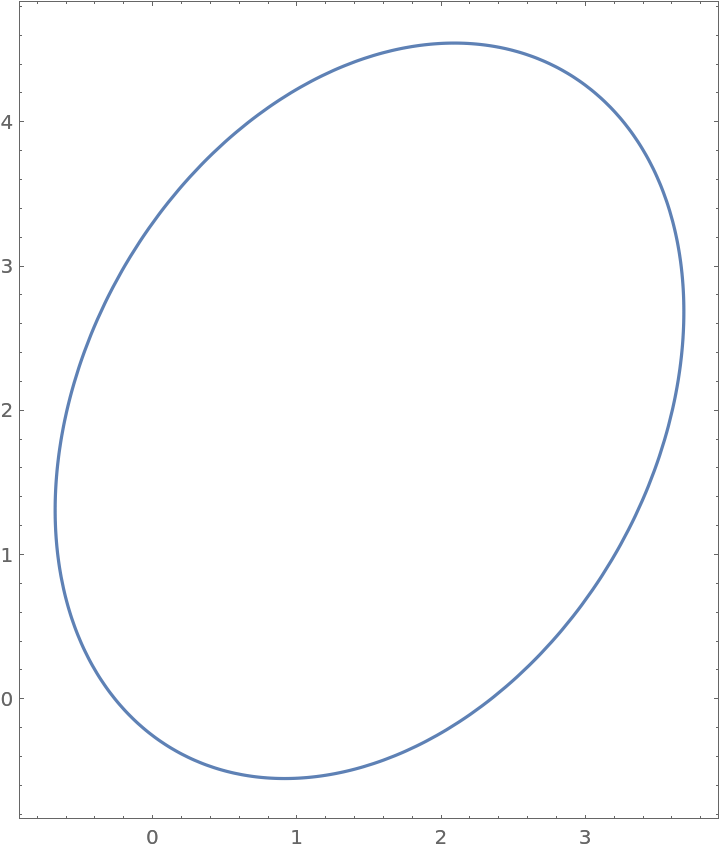The field of values for a Hermitian matrix is a line segment:

 In:=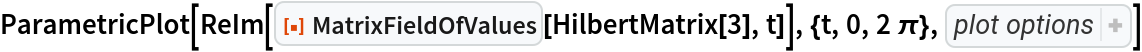Out=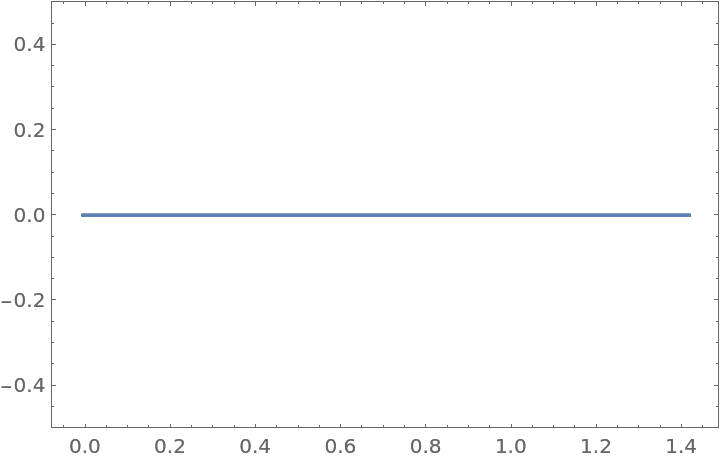The field of values for a diagonal matrix is the convex hull of its eigenvalues:

 In:=Out=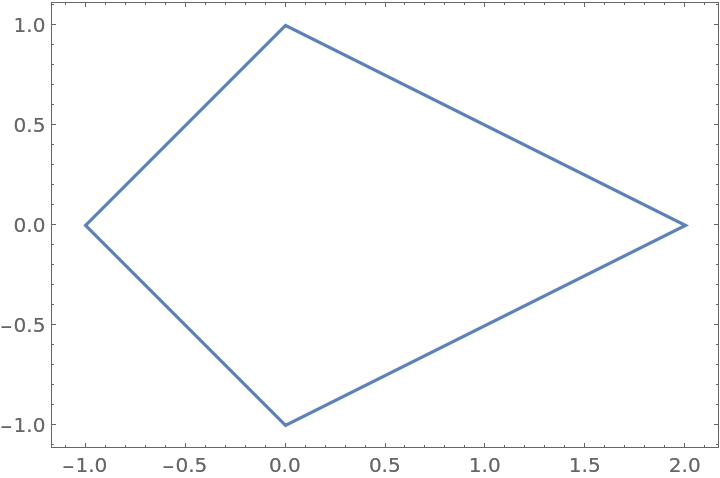## Version History

• 1.0.0 – 25 January 2021